﻿ Energiedichte für einen bestimmten Bereich - Mein MATLAB Forum - goMatlab.de# Mein MATLAB Forum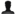Gast > Registrieren       Autologin?
﻿

 Studierende:

 Partner: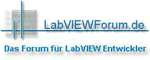Forum
 Option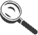Gehe zu: Forum auswählen Allgemeines----------------News zum ForumPro und Contra goMatlab-ForumMathworks News MATLAB - Allgemeine Fragen----------------Grafiken und PlotsGUIsProgrammierung"Suche Funktion für ..." FehlersuchePerformance-SteigerungObjektorientierte ProgrammierungToolboxenMatlab internRelease-FragenSonstige MATLAB FragenBugs MATLAB Simulink / Stateflow / Simscape----------------SimulinkStateflowSimscapeSimMechanics / SimDriveline / SimHydraulics / SimRF / SimElectronics / SimPowerSystemsBugs Simulink / Stateflow / Simscape MATLAB / Simulink / Stateflow - Spezielle Anwendungsgebiete----------------BildverarbeitungMathematikRegelungstechnikSignalverarbeitungCARNOT-Toolboxsonstige Anwendungsgebiete FAQ - MATLAB / Simulink / Stateflow----------------FAQFAQ Diskussion Scripte & Tutorials----------------Script-EckeTutorialsVideo-Tutorials Matlab-Alternativen----------------Scilab / Xcos (ehemals Scicos) ForumOctave-ForumFreeMat-Forum Sonstiges----------------Blog - Mein ProjektProgrammieraufträgeOff Topic Nutzertreffen----------------Allgemeine Diskussion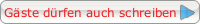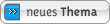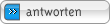## Energiedichte für einen bestimmten Bereich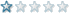Forum-AnfängerBeiträge: 30Anmeldedatum: 13.08.10Wohnort: DresdenVersion: ---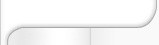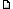Verfasst am: 26.08.2010, 13:27     Titel: Energiedichte für einen bestimmten Bereich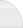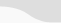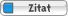Code: function [D] = D(M,Xmi,Xma,Ymi,Yma) % function to determine the energy density % With this function we get a Matrix, which contains only the Particles, % which are in the are we've determined through insert % Setting A as an empty matrix first A = []; % the length of the Matrix we've loadet before len=length(M); % searching all the particles which match with the area from Xmin-Xmax % and Ymin-Ymax for i = 1:len  if M(i,1)>=Xmi &  M(i,1)<=Xma   if M(i,2)>=Ymi & M(i,2)<=Yma   % Filling the empty Matrix A with the corresponding entrys      A = [A;M(i,:)];    end;   end;  end;   % now using the function to evaluate the Energy of the particles (only those in the new Matrix A)   % the vector x is a vector with all the data from all third collumns of your Matrix (gamma * beta x) x = A(:,3); % the vector x is a vector with all the data from all fourth collumns of your Matrix (gamma * beta y) y = A(:,4); % the vector x is a vector with all the data from all fivth collumns of your Matrix (gamma * beta z) z = A(:,5); % Evaluating the gamma for the formula: Ekin=(gamma-1)*m0*c^2 gamma = sqrt((x.^2)+(y.^2)+(z.^2)+1); % following wont be needet later, because constants will be loadet through % another Script and settet as global % Speed of light(in M/s, SI) global c = 2.99792458e+008 % Mass of a Proton(in kg, SI) global mp = 1.6726231e-024 % Mass of an Electron(in kg, SI) global me = 9.1093897e-031 % Mass of a Neutron(in kg, SI) global mn = 1.6749286e-024 % Getting Ekin for the formula: E=Ekin+Erest % "The total energy = the kinetic energy + the rest energy" Ekin = ((gamma-1).*(mp*(c^2))); % The total Energy: E = Ekin.+(mp*(c^2)); % summing of all the Energy of each particle Eg = sum(E); % getting the are we are currently in Area = ((Xma-Xmi)*(Yma-Ymi)); % finally: evaluating the energy density: D = (Eg/Area);     end     Funktion ohne Link?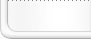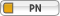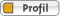Einstellungen und Berechtigungen Beiträge der letzten Zeit anzeigen: Alle Beiträge1 Tag7 Tage2 Wochen1 Monat3 Monate6 Monate1 Jahr Die ältesten zuerstDie neusten zuerst Du kannst Beiträge in dieses Forum schreiben.Du kannst auf Beiträge in diesem Forum antworten.Du kannst deine Beiträge in diesem Forum nicht bearbeiten.Du kannst deine Beiträge in diesem Forum nicht löschen.Du kannst an Umfragen in diesem Forum nicht mitmachen.Du kannst Dateien in diesem Forum postenDu kannst Dateien in diesem Forum herunterladen
 .
﻿

Impressum  | Nutzungsbedingungen  | Datenschutz  | Werbung/Mediadaten | Studentenversion | FAQ |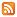RSS

Copyright © 2007 - 2023 goMatlab.de | Dies ist keine offizielle Website der Firma The Mathworks
Partner: LabVIEWforum.de

MATLAB, Simulink, Stateflow, Handle Graphics, Real-Time Workshop, SimBiology, SimHydraulics, SimEvents, and xPC TargetBox are registered trademarks and The MathWorks, the L-shaped membrane logo, and Embedded MATLAB are trademarks of The MathWorks, Inc.